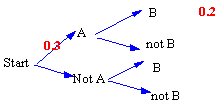### CFA Practice Question

There are 985 practice questions for this topic.

### CFA Practice Question

For the tree diagram shown at the right,P(B | A) = ______

A. 1/3
B. 3/7
C. 2/3

From the tree diagram, we know that 0.3 times P(B | A) = 0.2. Now, 0.3x = 0.2 yields x = 0.2/0.3 = 2/3. Thus, P(B | A) = 2/3.

User Comment
gth763s P(B|A)=0.2, P(AB)=.06
mordja No P(B|A) = 2/3

P (AB) = 0.2
fedha Here is how I solved the problem

P(B|A) = P(BA)/P(A)
P(B|A) = 0.2/0.3
P(B|A) = 0.667 ~ 2/3
Scc0813 Why is 0.2 P(AB) and not P(B|A)?
choas69 P(AB) = P(A) x P(B/A)
0.2 = 0.3 x P(B/A)
P(B/A)= 0.2/0.3
choas69 scc 0.2 is the result of A and B together not B given A